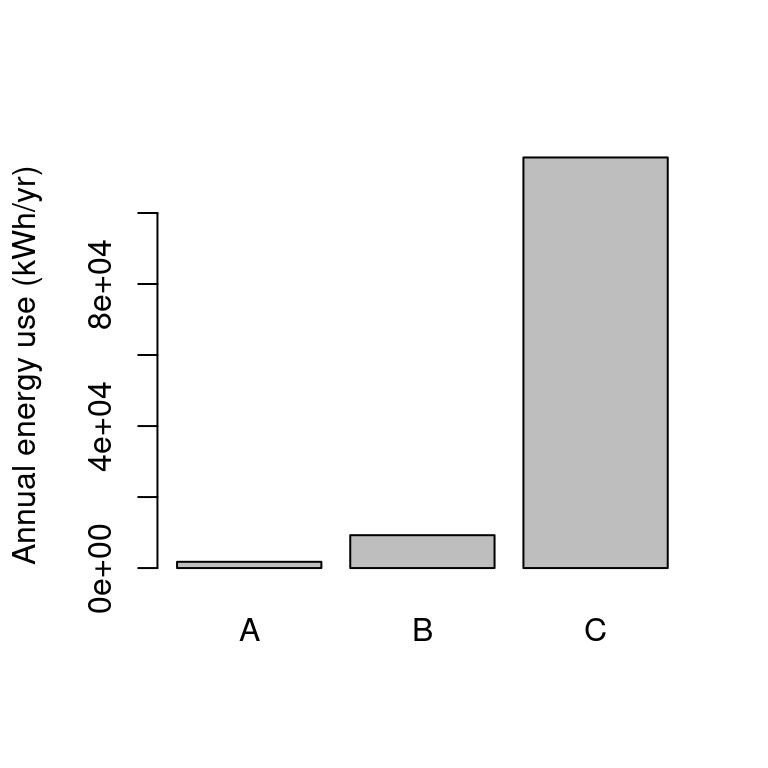### Energy expenditure of computational research in context

There have been a few papers recently on “green computing”, and changes we can make to ensure our work is more sustainable. The most important aspect to this is the overuse of energy in conducting our analysis, especially if the energy is derived from burning fossil fuels. What I want to do here is to put in context the energy expenditure of research computing systems by comparing it to other energy expenditures such as travel and transport.

## Energy consumption of a workstation or small server

In order to quantify the energy expenditure of a compute system, we need to make some assumptions.

We will assume that for bioinformatics, the CPU is the main consumer of power, is working at 50% of capacity.

• AMD Ryzen 9 5950X (16 cores / 32 threads) Maximum power consumption = 142 W /2 = 71 W source
• High end motherboard: 75 W.
• RAM stick: 3 W * 8 = 24 W
• Mid range graphics card idle = 12 W
• HDD Storage: 9 W * 2 = 18 W
Total: 71 + 75 + 24 + 12 + 18 = 200 W

So a workstation, or small server with 32 threads and 64-128 GB RAM will consume about 200 W at 50% CPU utilisation.

Multiply this by the number of hours in the year (8766 hrs) and you get 1753.2 kWh (or 54.8 kWh per thread) consumed per year.

## Commuting by car

It is stated that 100 km traveled by mid size car (Volkswagen Passat) costs 83.5 kWh/100km. So a 30 km commute will consume 25 kWh each way. Attending work 150 days per year will cost 25 * 150 * 2 = 7,500 kWh per year.

So 1 person commuting by car 150 days per year is the same as running 137 CPU threads, or 4 small servers for a year.

## Overseas travel

Conferences are a key part of science these days, so it is fair to calculate the energy cost of airline travel.

We will calculate a travel from New York to Berlin, which is 7,934 km return.

Fuel consumption per passenger is approx 3.5 L/100 km.

So a return flight NY-Berlin will consume: 7934 km * 3.5 L / 100 km = 277.7 L fuel.

Energy content of aviation fuel is 690 MJ/L, so the energy expenditure is 690 MJ/L * 277.7 L = 191.6 GJ

This comes to 53,200 kWh.

This does not include the energy expenditure to extract, refine and transport the fuel itself.

So the international conference trip is the same as running 971 CPU threads, or ~30 small servers for a whole year.

## Conclusion

While power consumption from computational research could be an environmental concern, it is important to put it in context.

If researcher A uses one server at 50% CPU load, and works remotely, they will expends 1,753 kWh/yr.

If reseracher B uses one server and commutes 150 days er year by car, that is 9,253 kWh/yr.

If researcher C uses one server and commutes by car 150 days per year and makes two intercontinental return flights annually, they will use 115,653 kWh.Therefore, for typical workloads involving five servers or less, an individual’s travel behaviour is a much greater consideration than the energy cost of computing.

The energy consumption of air travel is substantial and we should strongly discourage intercontinental conference travel.

Lastly, the environmental impact of air travel is likely much worse than stated here due to the environmental damages caused by fuel extraction, refining and transport, and the fact that fixed-grid energy is more likely to originate from renewable sources like solar and wind, whereas transport fuel combustion results directly in emissions.

Source: https://github.com/markziemann/energy/